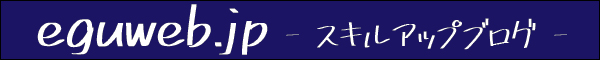# What is NPV (Net Present Value)?

## What is NPV (Net Present Value)?

NPV (Net Present Value) is how much profit you can get from your investment. It is an index that shows

It refers to the amount obtained by subtracting the initial cost (investment amount) from the total present value of the cash flow generated by the project of the investment target business .

The higher the NPV, the more value you create . Therefore, NPV is one of the important indicators used for business investment decisions.

First of all, regarding PV of NPV, this is called ” present value “.

What is the current value of the money you plan to get in the future? is an index.

## How to calculate NPV (Net Present Value)

The formula for calculating the net present value using the NPV method (net present value method) is as follows.

Net Present Value = Present Value – Investment Amount

If the present value is 10 million yen and the investment amount is 6 million yen, the net present value = 1,000 – 6 million = 4 million yen.

NPV (Net Present Value) can be obtained by the following calculation method.

1 million yen x (1+0.01) = 1.01 million yen

## summary

This time it was the basics of “IRR (Internal Rate of Return)”.

The value of discount rate r when NPV = 0 is called “IRR (internal rate of return)”. We will talk about this on another page.